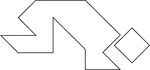### Diver

Tangrams, invented by the Chinese, are used to develop geometric thinking and spatial sense. Seven figures…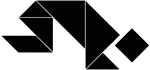### Diver

Tangrams, invented by the Chinese, are used to develop geometric thinking and spatial sense. Seven figures…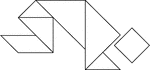### Diver

Tangrams, invented by the Chinese, are used to develop geometric thinking and spatial sense. Seven figures…### Hexagon And Triangle Inscribed In A Dodecagon

Illustration of a regular hexagon and an equilateral triangle inscribed in a regular dodecagon. This…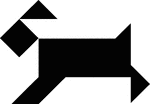### Dog

Tangrams, invented by the Chinese, are used to develop geometric thinking and spatial sense. Seven figures…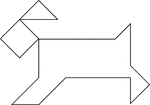### Dog

Tangrams, invented by the Chinese, are used to develop geometric thinking and spatial sense. Seven figures…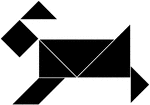### Dog

Tangrams, invented by the Chinese, are used to develop geometric thinking and spatial sense. Seven figures…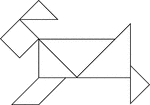### Dog

Tangrams, invented by the Chinese, are used to develop geometric thinking and spatial sense. Seven figures…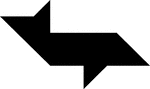### Dolphin

Tangrams, invented by the Chinese, are used to develop geometric thinking and spatial sense. Seven figures…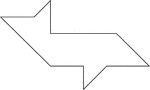### Dolphin

Tangrams, invented by the Chinese, are used to develop geometric thinking and spatial sense. Seven figures…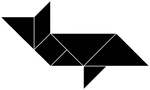### Dolphin

Tangrams, invented by the Chinese, are used to develop geometric thinking and spatial sense. Seven figures…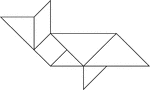### Dolphin

Tangrams, invented by the Chinese, are used to develop geometric thinking and spatial sense. Seven figures…### doodad

three small leaves together forming a geometrical shape.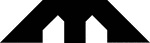### Double Arch

Tangrams, invented by the Chinese, are used to develop geometric thinking and spatial sense. Seven figures…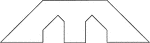### Double Arch

Tangrams, invented by the Chinese, are used to develop geometric thinking and spatial sense. Seven figures…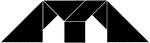### Double Arch

Tangrams, invented by the Chinese, are used to develop geometric thinking and spatial sense. Seven figures…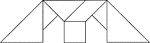### Double Arch

Tangrams, invented by the Chinese, are used to develop geometric thinking and spatial sense. Seven figures…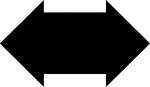### Double Arrow

Tangrams, invented by the Chinese, are used to develop geometric thinking and spatial sense. Seven figures…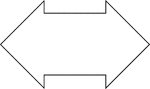### Double Arrow

Tangrams, invented by the Chinese, are used to develop geometric thinking and spatial sense. Seven figures…### Double Arrow

Tangrams, invented by the Chinese, are used to develop geometric thinking and spatial sense. Seven figures…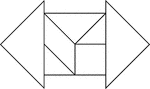### Double Arrow

Tangrams, invented by the Chinese, are used to develop geometric thinking and spatial sense. Seven figures…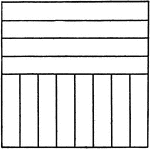### Drawing Exercise for T-square, Triangle, and Scale

"Through the center of the space draw a horizontal and a vertical line, measuring on these lines as…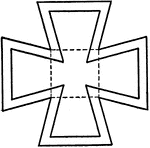### Drawing Maltese Cross using T-square, Spacers, and Triangles

"Draw three-inch square and one-inch square. From the corners of inner square draw lines to outer square…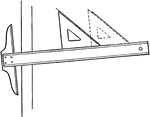### Drawing Parallel Lines Using T-square and Triangle

Parallel lines are drawn by holding the triangle against the T-square at an angle, or another triangle,…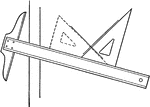### Drawing Perpendicular Lines Using T-square and Triangle

Perpendicular lines are drawn by fitting the hypothenuse of the triangle to the T-square at an angle,…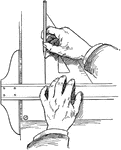### Drawing Vertical Lines Using a T-square and a Triangle

When drawing vertical lines, the blade of the T-square is held with the thumb while the fingers adjusting…### Duck

Tangrams, invented by the Chinese, are used to develop geometric thinking and spatial sense. Seven figures…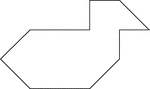### Duck

Tangrams, invented by the Chinese, are used to develop geometric thinking and spatial sense. Seven figures…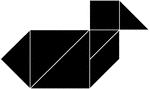### Duck

Tangrams, invented by the Chinese, are used to develop geometric thinking and spatial sense. Seven figures…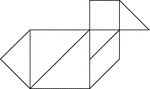### Duck

Tangrams, invented by the Chinese, are used to develop geometric thinking and spatial sense. Seven figures…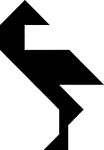### Egret Facing Backward

Tangrams, invented by the Chinese, are used to develop geometric thinking and spatial sense. Seven figures…### Egret Facing Backward

Tangrams, invented by the Chinese, are used to develop geometric thinking and spatial sense. Seven figures…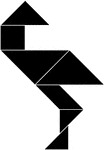### Egret Facing Backward

Tangrams, invented by the Chinese, are used to develop geometric thinking and spatial sense. Seven figures…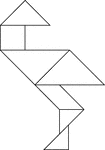### Egret Facing Backward

Tangrams, invented by the Chinese, are used to develop geometric thinking and spatial sense. Seven figures…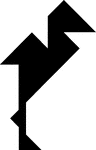### Egret Facing Forward

Tangrams, invented by the Chinese, are used to develop geometric thinking and spatial sense. Seven figures…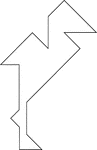### Egret Facing Forward

Tangrams, invented by the Chinese, are used to develop geometric thinking and spatial sense. Seven figures…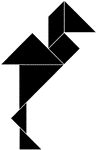### Egret Facing Forward

Tangrams, invented by the Chinese, are used to develop geometric thinking and spatial sense. Seven figures…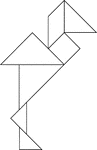### Egret Facing Forward

Tangrams, invented by the Chinese, are used to develop geometric thinking and spatial sense. Seven figures…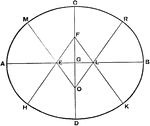### Ellipse Fifth Method

Draftsman's fifth method for drawing an ellipse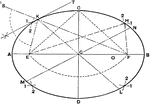### Ellipse First Method

Draftsman's first method for creating an ellipse### Centers of Equilateral Triangle

Illustration of an equilateral triangle that shows both the centroid (where the medians of the sides…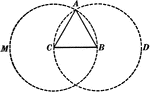### Construction Of Equilateral Triangle

Illustration used to show how to construct an equilateral triangle, with a given line as a side.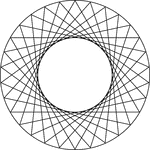### 10 Congruent Rotated Equilateral Triangles

Illustration of 10 congruent equilateral triangles that have the same center. Each triangle has been…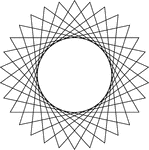### 10 Congruent Rotated Equilateral Triangles

Illustration of 10 congruent equilateral triangles that have the same center. Each triangle has been…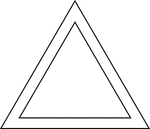### 2 Concentric Equilateral Triangles

Illustration of 2 concentric equilateral triangles.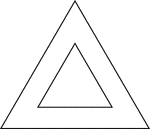### 2 Concentric Equilateral Triangles

Illustration of 2 concentric equilateral triangles.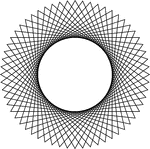### 20 Congruent Rotated Equilateral Triangles

Illustration of 20 congruent equilateral triangles that have the same center. Each triangle has been…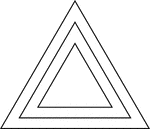### 3 Concentric Equilateral Triangles

Illustration of 3 concentric equilateral triangles that are equally spaced.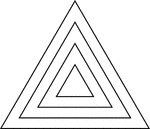### 4 Concentric Equilateral Triangles

Illustration of 4 concentric equilateral triangles that are equally spaced.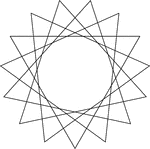### 5 Congruent Rotated Equilateral Triangles

Illustration of 5 congruent equilateral triangles that have the same center. Each triangle has been…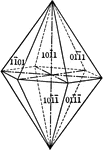### Pyramid of the first order

"This form consists of twelve isoceles triangular faces, each of which intersects two of the horizontal…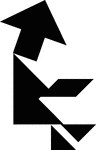### Fisherman

Tangrams, invented by the Chinese, are used to develop geometric thinking and spatial sense. Seven figures…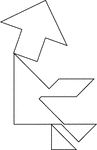### Fisherman

Tangrams, invented by the Chinese, are used to develop geometric thinking and spatial sense. Seven figures…### Fisherman

Tangrams, invented by the Chinese, are used to develop geometric thinking and spatial sense. Seven figures…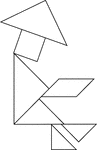### Fisherman

Tangrams, invented by the Chinese, are used to develop geometric thinking and spatial sense. Seven figures…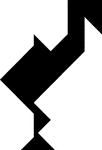### Flamingo

Tangrams, invented by the Chinese, are used to develop geometric thinking and spatial sense. Seven figures…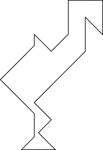### Flamingo

Tangrams, invented by the Chinese, are used to develop geometric thinking and spatial sense. Seven figures…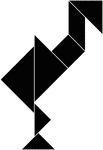### Flamingo

Tangrams, invented by the Chinese, are used to develop geometric thinking and spatial sense. Seven figures…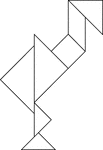### Flamingo

Tangrams, invented by the Chinese, are used to develop geometric thinking and spatial sense. Seven figures…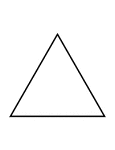### Flashcard of a polygon with three equal sides

A flashcard featuring an illustration of a polygon with three equal sides